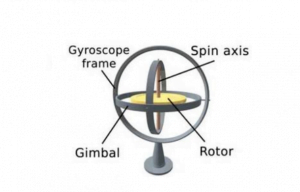Application

# How does Inertial Positioning Solve the Problem of Gyroscope Drift and Magnetic Field Interference?In order to solve the problems of integral error and magnetic field interference of gyroscope and electronic compass

in attitude calculation of navigation system, a fusion algorithm of Kalman filter and complementary filter was proposed. First, the electronic compass and gyroscope are obtained through Kalman filter to obtain the optimal estimated quaternion. Then, the complementary filtering algorithm is used to compensate the drift of gyroscope to obtain the corrected quaternion. Then, the obtained quaternion and Kalman filter are used to obtain the optimal estimated quaternion, and the second optimal estimation of the quaternion is conducted through Kalman filter.Then output attitude Angle.The results of the proposed algorithm, the complementary filtering algorithm and the non-filtering algorithm are compared in the experiment.Experiments show that the algorithm can not only effectively solve the divergence of azimuth error, but also effectively solve the magnetic field interference, and achieve high precision azimuth output。

With the development of miniaturized inertial devices represented by MEMS (Micro-Electromechanical Systems) sensors, the inertial positioning technology based on strapdown inertial navigation principle and MEMS sensors is increasingly paid attention to.Especially in indoor, underground, mine, underwater, battlefield and other occasions where satellite signals are difficult to receive .In view of the above problems, the electronic compass is often used to correct the gyro. In the indoor, underground, mine, underwater and other processes, the magnetometer is more prone to interference, resulting in greater deviation of orientation.To solve the problems of magnetometer vulnerable to interference and gyro integral drift, there have been numerous fusion algorithms, such as Kalman filter, untracked Kalman filter (UKF), extended Kalman filter (EKF), etc. [2-4]. These filtering methods need to establish accurate state equation and observation equation. There is another filtering algorithm that extends on the basis of complementary filtering, such as classical complementary filtering and complementary filtering algorithm based on gradient descent method [3-6]. However, the accuracy of this filtering algorithm is not high.Face these problems, this paper proposes a inertial positioning algorithm of Kalman filtering and complementary filtering fusion, the algorithm in the design of Kalman filter, the accelerometer and magnetometer fusion of quaternion as observed value, using the gyroscope of quaternion as a status value, through the data fusion filtering, complete the quaternion optimal estimate for the first time,For the gyro drift problem, the designed complementary filter is used to compensate the gyro drift, and the corrected angular velocity is obtained, and then the continuously updated quaternion after correction is obtained. Then, the optimal estimation quaternion completed at the first time is estimated through the second Kalman filter, and then the high-precision attitude angle is output.

Gyroscope in the navigation calculating integral deviation and magnetometer susceptible to interference problems, put forward a kind of using Kalman filter and the localization algorithm of inertia of the integration of complementary filter using Kalman filter for the optimal quaternion and the angular velocity gyroscope to make use of the complementary filter correction, through the correction of the angular velocity of the quaternion update,The quaternion after two corrections is obtained through Kalman filter to obtain the optimal quaternion.It can be seen from the experimental results that this method can not only solve the gyro drift problem well, but also effectively suppress the interference problem of the magnetometer, which can accurately calibrate the opposite position and realize the stable output of the attitude Angle with high precision.